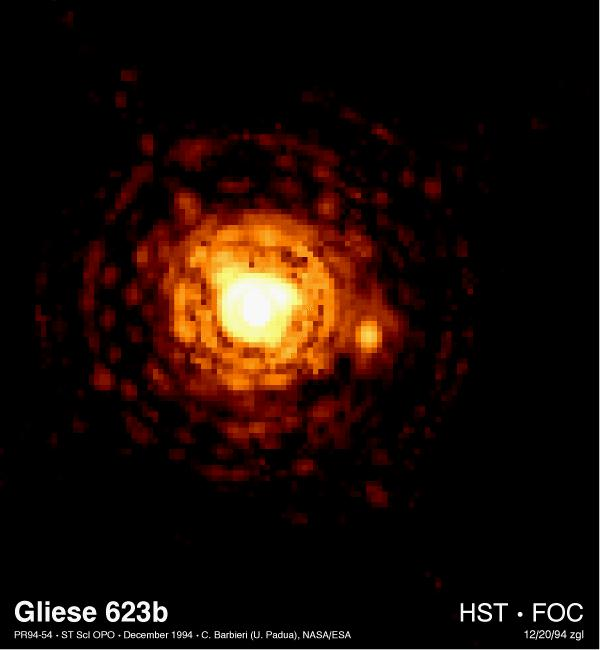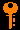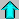# Lecture 5 - Visual Binary Systems (1/26/99)Mechanics --- | --- Binaries IIChapter 1-5, 12-2 (ZG4)
Prelude P1, P2 (ZG4)

Notes:

pages 16 - 19

HST FOC image of binary star Gliese 623. The companion is Gl623b, with a mass of 0.1 Msun, in a 2AU orbit with a 4 year period around the brighter primary Gl623a. (Courtesy STSCI)
Key Question:## Investigations:

1. Orbital Mechanics continued
• Why are energy and angular momentum integrals of the motion?
• How do energy and angular momentum generalize to systems with more than 2 bodies?
• What is the virial theorem?
• How does the total energy determine the semi-major axis of the orbit?
• How does the total angular momentum determine the eccentricity and relate to Kepler's 2nd Law?
• What is Kepler's 3rd Law in its final center-of-mass form?
• What two equations give the total mass and mass ratio for the binary system?
• What are the limitations for using visual binary systems to determine masses for a large number of stars?
2. Visual Binaries - A Primer
• What are periastron and apastron?
• What are the primary and secondary in a binary?
• What is the line of apsides and how does its inclination affect how the orbit appears on the sky?
• How do you determine the inclination of a visual binary orbit?
• Why must a binary orbit be confined to a plane?
• What input do you need to determine the mass using Kepler's 3rd Law?
3. Example (see homework 1): Sirius A & B - Masses and Luminosities
• System Data -

 Period: 49.94 years Parallax: 0.377" Apparent Magnitudes (bol): -1.55, +5.69 Apparent Semi-Major Axis: 7.62" Ratio of Orbits a_A/a_B: 0.466 Inclination: ~0

• What is the distance to Sirius in parsecs?
• What are the absolute bolometric magnitudes for Sirius A,B?
• What are the luminosities of Sirius A,B?
• What is the orbital semi-major axis in AU?
• What is the mass ratio m_A/m_B?
• What is the total mass M = m_A + m_B?
• What are the individual masses m_A, m_B?
• What are the mass-to-light ratios M/L of Sirius A,B?
• How do Sirius A, B compare with the Sun?

## Binary Stars with HST:

Some binary stars and related objects reported in Hubble Space Telescope press releases include:Prev Lecture ---Next Lecture ---Astr12 Index ---Astr12 Home

smyers@nrao.edu Steven T. Myers